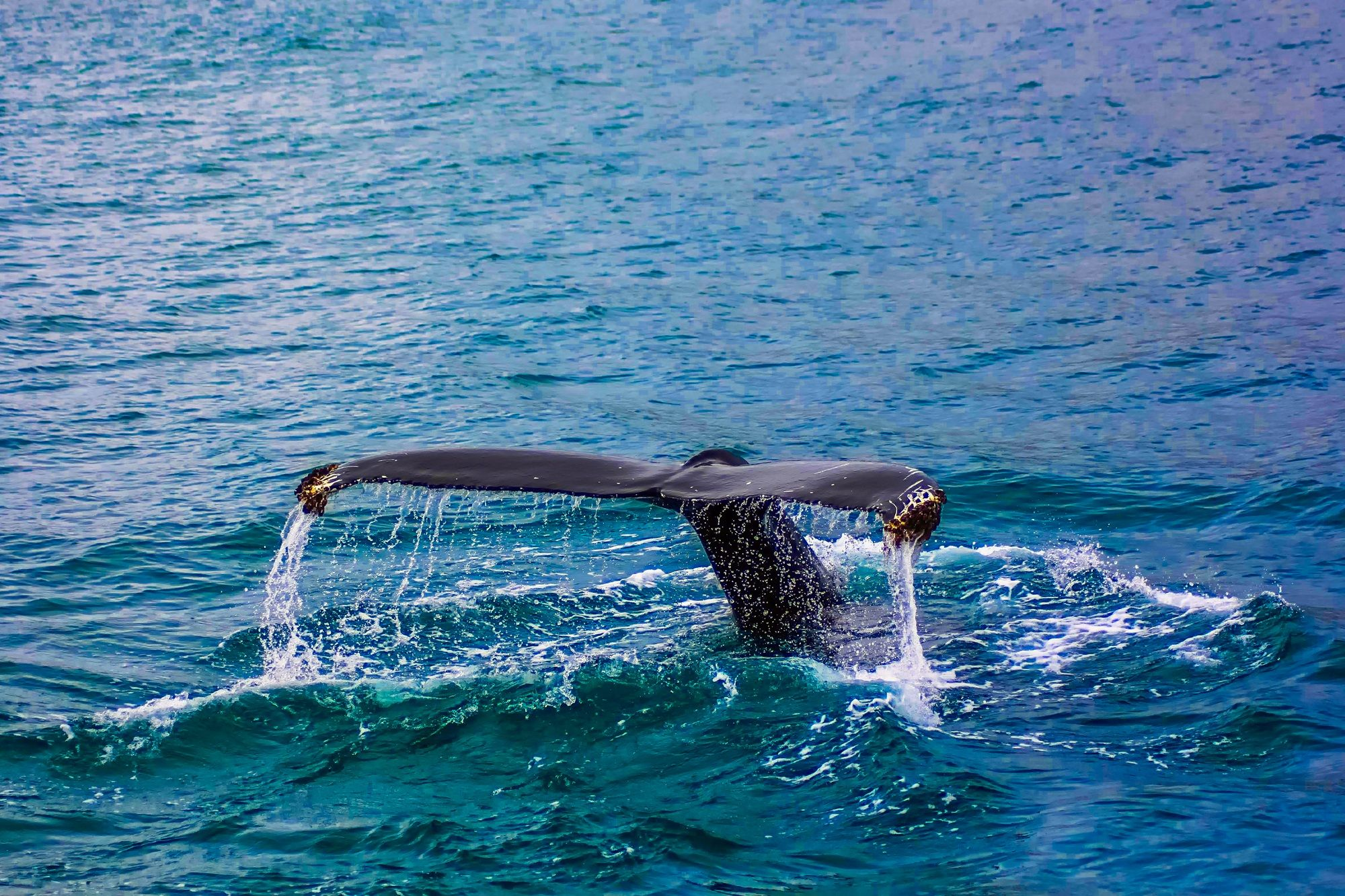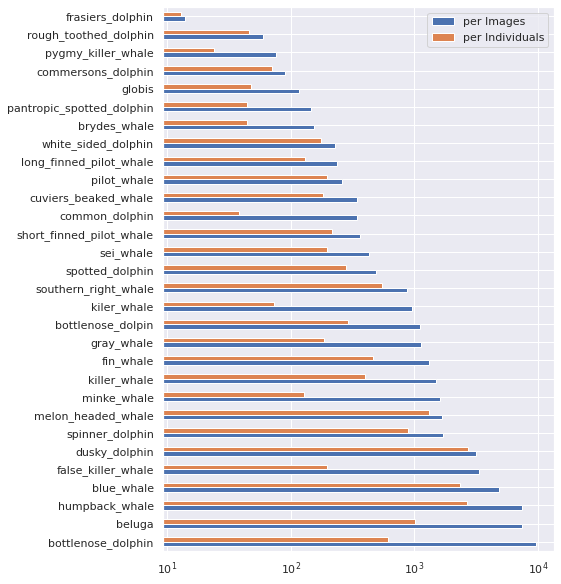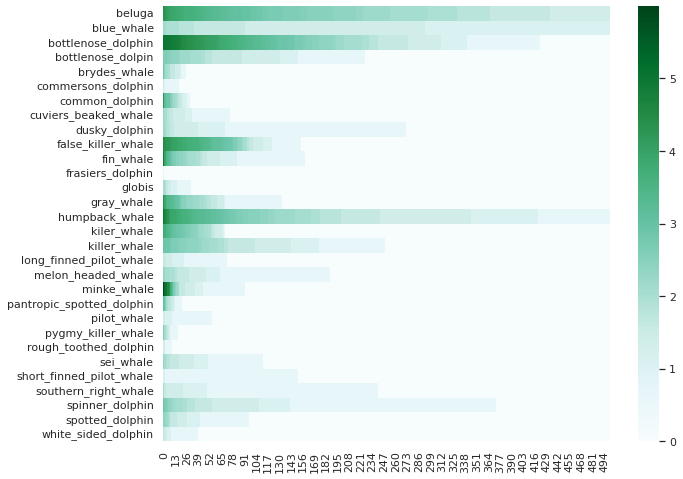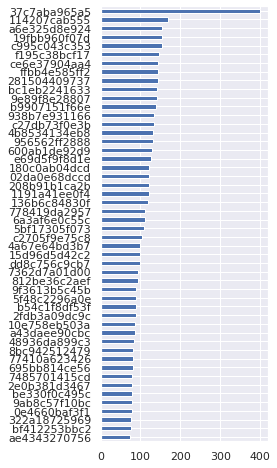In this Notebook article, you will learn how to write a custom training loop in pure PyTorch, create custom torch `Dataset` class, compute metrics for model performance, and Scale the Training on any hardware like GPU, TPU, IPU or Distributed Training with LightningLite.

Checkout the original Kaggle Notebook here.# 🕵 Explore the provided data

(EDA is taken from Notebook of Jirka)

```!ls -l /kaggle/input/happy-whale-and-dolphin

PATH_DATASET = "/kaggle/input/happy-whale-and-dolphin"
```
```total 4668
-rw-r--r-- 1 nobody nogroup 2404234 Feb  1 16:45 sample_submission.csv
drwxr-xr-x 2 nobody nogroup       0 Feb  1 16:47 test_images
-rw-r--r-- 1 nobody nogroup 2371769 Feb  1 16:47 train.csv
drwxr-xr-x 2 nobody nogroup       0 Feb  1 16:51 train_images
```

```import os
import pandas as pd
import seaborn as sn
import matplotlib.pyplot as plt

sn.set()

print(f"Dataset size: {len(df_train)}")
print(f"Unique ids: {len(df_train['individual_id'].unique())}")
```
image species individual_id
1 000562241d384d.jpg humpback_whale 1a71fbb72250
2 0007c33415ce37.jpg false_killer_whale 60008f293a2b
3 0007d9bca26a99.jpg bottlenose_dolphin 4b00fe572063
4 00087baf5cef7a.jpg humpback_whale 8e5253662392
```Dataset size: 51033
Unique ids: 15587
```

Lets see how many speaced we have in the database...

```counts_imgs = df_train["species"].value_counts()
counts_inds = df_train.drop_duplicates("individual_id")["species"].value_counts()

ax = pd.concat({"per Images": counts_imgs, "per Individuals": counts_inds}, axis=1).plot.barh(grid=True, figsize=(7, 10))
ax.set_xscale('log')
```And compare they with unique individuals...

Note: that the counts are in log scale

```import numpy as np
from pprint import pprint

species_individuals = {}
for name, dfg in df_train.groupby("species"):
species_individuals[name] = dfg["individual_id"].value_counts()

si_max = max(list(map(len, species_individuals.values())))
si = {n:  * si_max for n in species_individuals}
for n, counts in species_individuals.items():
si[n][:len(counts)] = list(np.log(counts))
si = pd.DataFrame(si)
```
```import seaborn as sn

fig = plt.figure(figsize=(10, 8))
ax = sn.heatmap(si[:500].T, cmap="BuGn", ax=fig.gca())
```And see the top individulas

```ax = df_train["individual_id"].value_counts(ascending=True)[-50:].plot.barh(figsize=(3, 8), grid=True)  # ascending=True
```## Browse some images

```nb_species = len(df_train["species"].unique())
fig, axarr = plt.subplots(ncols=5, nrows=nb_species, figsize=(12, nb_species * 2))

for i, (name, dfg) in enumerate(df_train.groupby("species")):
axarr[i, 0].set_title(name)
for j, (_, row) in enumerate(dfg[:5].iterrows()):
im_path = os.path.join(PATH_DATASET, "train_images", row["image"])